## More problems with the XIRR function in EXCEL

Feb 3

Written by: Charles Flock
2/3/2010 6:27 PMAs a follow up to our previous blogs documenting problems with the XIRR function in EXCEL and what an EXCEL XIRR of 2.98023E-09 really means.
Recently we received a support request from a customer wanting to know why EXCEL’s XIRR function was returning a different result from the XLeratorDB XIRR_q function. As is usually the case with these things, there were many cash flows, lots of switching of signs, and a large change in the cumulative cash flow from the initial cash flow to the final one. We dutifully downloaded the data, and EXCEL produced an XIRR value of 2.98023E-09 (which is approximately zero). I thought to myself, that number looks very familiar and quickly created a test scenario in EXCEL that reproduced that result with far fewer cash flows.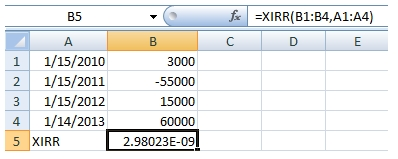Before we go any further, let’s review what the XIRR calculation does. You can find the Microsoft documentation
here. In simple terms, XIRR is trying to find a value, R, such that when R is passed to the XNPV calculation with the same data, it will return approximately zero. To check the value that EXCEL calculated, we can simply take the XIRR value that was calculated and pass it into the XNPV function.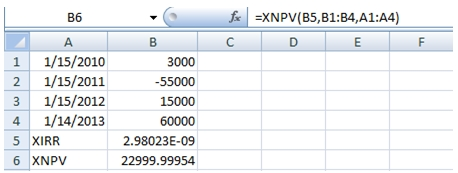As you can see, XNPV calculates a value of almost 23,000, or almost exactly the sum of the cash flows, which is what we should expect with a discount rate of almost zero. At a rate of zero, the sum of the discounted cash flows and the sum of the cash flows will be equal.
One thing that we can do is determine what the IRR value is.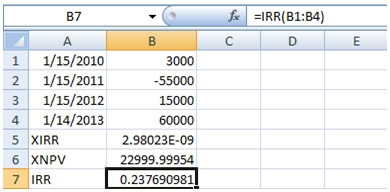Since the cash flows occur every 365 days, we would expect the XIRR value and the IRR values to be similar. The IRR value is about 23.77%very different from the XIRR value.
What would happen if we used Excel's Solver to come up with value?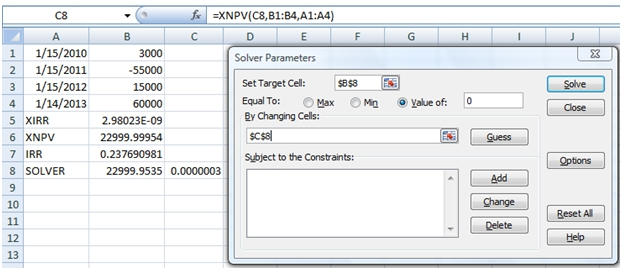This produces the following result.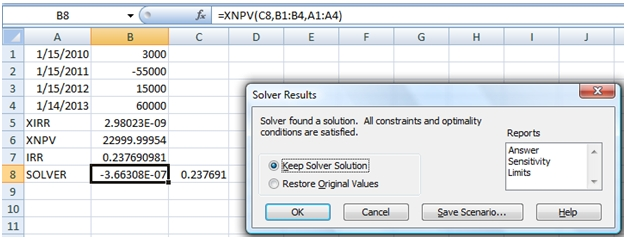The Solver solution, .237691, is extremely close to the IRR value and when used in the XNPV function returns a value pretty close to zero. It seems clear that the EXCEL XIRR solution needs to be thrown out.
What happens in XLeratorDB? We run the following SQL:
SELECT wct.XIRR_q(REPLACE('SELECT 3000,''01/15/2010''
-55000,''01/15/2011''
15000,''01/15/2012''
60000,''01/14/2013''', CHAR(10), 'UNION ALL SELECT ')
,NULL)

producing the following result.

----------------------
0.237690981047194

(1 row(s) affected)

As you can plainly see, this number is very similar to the answers produced by Solver and by Excel’s IRR function, which is to be expected. Let’s take this result and run it through the EXCEL XNPV function.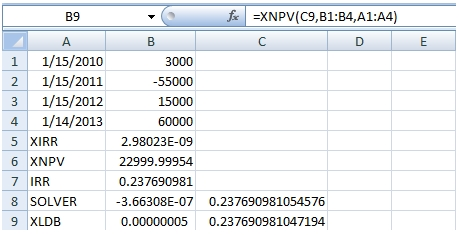It seems clear that the XLeratorDB function returns a value, R, which satisfies the requirement of producing an XNPV result of approximately zero. Here’s how that would look in SQL Server:

SELECT wct.XNPV_q(wct.XIRR_q(REPLACE('SELECT 3000,''01/15/2010''
-55000,''01/15/2011''
15000,''01/15/2012''
60000,''01/14/2013''', CHAR(10), 'UNION ALL SELECT ')
,NULL),REPLACE('SELECT 3000,''01/15/2010''
-55000,''01/15/2011''
15000,''01/15/2012''
60000,''01/14/2013''', CHAR(10), 'UNION ALL SELECT '))

This produces the following result

----------------------
5.1648385124281E-08

(1 row(s) affected)

In a previous
blog we reported that under certain situations the XIRR function is sensitive to the order of data; that it will return different results for the same data depending upon how the data are sorted. Now there are situations where the result is actually wrong. In fact, we have discovered that any time the EXCEL XIRR function returns a value of 2.98023E-09, you should view it with suspicion. This is actually quite insidious, because when XIRR cannot resolve Excel generates the #NUM! error value. Here it has generated an erroneous value, which looks OK but cannot be relied upon.

The solution, clearly, is to stop using EXCEL for important calculations like this and start doing this in SQL Server using XLeratorDB.

You may also watch a video of this demonstration of Excel's XIRR problem.

Tags:
Categories:
Location: BlogsThe WestClinTech Blog

## Search Blogs

 KeywordsPhrase

## Blog Archives

Archive
 < September 2023 >
SunMonTueWedThuFriSat
272829303112
3456789
10111213141516
17181920212223
24252627282930
1234567
Monthly
Go

### SupportCopyright 2008-2023 Westclintech LLC         Privacy Policy        Terms of Service